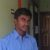You are here: Home > Competition Exam > Olympiad
All posts from

# math.iitb.ac.in Mathematics Olympiad MO 2016 : IIT Bombay

Organisation : IIT Bombay
Announcement : Mathematics Olympiad MO 2016

Mathematics Olympiad is a talent search event involving schools and colleges from all over India conducted by Mathematics Association of IIT Bombay.

This year the Mathematics Association is organizing the 34th Mathematics Olympiad. This is a platform for students to challenge themselves and test their Mathematical knowledge and skills. The goal of Mathematics Olympiad is to stimulate enthusiasm and love for mathematics, to strengthen mathematical intuition, foster mathematical creativity and provide satisfaction, joy and thrill of meeting challenges.

It is a great opportunity for the participating students to experience the atmosphere of IIT Bombay, which is one of the premium academic institutions of the country. The Olympiad is overseen by some of the most renowned professors of the country, who are faculty at the Mathematics Department of IIT Bombay.

Original Problems
Enhancing problem solving skills
Creating a positive environment
Exciting gifts and certificates for all

Eligibility :
Level 1 : Students from standard 8th, 9th and 10th can appear for the Level 1 examination.
Level 2 : Students from standard 11th and 12th are eligible for the Level 2 examination.
Level 3 : Undergraduate students (upto 3rd year) are eligible for level 3 examination.

Registration will be open from : 1st November 2015 till 1st January 2016
Date : 24th January 2016
Venue : IIT Bombay

All one needs to crack the Olympiad is common sense and problem solving skills.
Here is a rough list of topics in which one might expect questions.
It is by no means a comprehensive one. It is meant to give the students an idea of what questions might be asked.

Level I :
Arithmetic : Basic Arithmetic, Ratio and Proportion, Profit and Loss, Time and Distance, Time and Work
Algebra : Basic Algebra, Simultaneous/Quadratic Equations, Logarithms, Surds, Indices
Geometry : Basic Geometry, Area and Volume, Mensuration, Triangle, Quadrilateral, Circle, Trigonometry
Number Theory : Integers, Permutations and Combinations, Basic Probability, Divisibility, Counting, Sequences and Series

Level II :
Arithmetic : Basic Arithmetic, Ratio and Proportion, Profit and Loss, Time and Distance, Time and Work
Algebra : Basic Algebra, Polynomials and Equations, Logarithm, Surds, Indices, Complex Numbers
Geometry : Basic Geometry, Area and Volume, Mensuration, Polygons, Trigonometry, 2-Dimensional and 3-Dimensional Coordinate Geometry
Number Theory : Permutations and Combinations, Basic Probability, Divisibility, Counting, Sequences and Series, Mathematical Induction
Calculus : Functions, Differentiability, Differentiation, Limits, Continuity, Integration, Applications of Calculus

Level III :
Analysis : Real Analysis, Complex Analysis, Series and Sequences of Functions, Power Series, Metric Spaces
Algebra : Basic Algebra, Polynomials, Theory of Equations, Linear Algebra, System of Linear Equations, Group Theory
Geometry : Basic Geometry, Area and Volume, Mensuration, Polygons, Trigonometry, 2-Dimensional and 3-Dimensional Coordinate Geometry
Number Theory : Basic Number Theory, Permutations and Combinations, Divisibility, Counting
Calculus : Functions, Differentiability, Differentiation, Limits, Continuity, Integration, Aplications of Calculus, Ordinary Differential Equations
Basic Arithmetic, Probability and Statistics

Have any query, question or suggestions for us ? Feel free to contact us. We would be happy to hear from you
Mathematics Association, IIT Bombay, 400076
assoc.iitb@gmail.com
assoc@math.iitb.ac.in

1.I am a class 8 student. I am preparing myself for IMO level 2 RMO(regional mathematics Olympiad). What should I do?

2.How to take episilon and delta in limits and continuity proofs?

3.How to find result individually?

1.You can check the result by searching your roll number in the pdf which contains list of selected students.